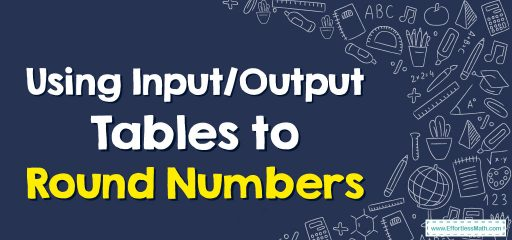# Using Input/Output Tables to Round Numbers

Input is the figure in the 1st column of the table. That’s the number you begin with in order to follow this rule if the rule has been given.

Output, in contrast, is the end number of the solution to the mathematics equation. The rule is that the mathematics operation has to be used in order to get the right sets of numbers for your input-output table.## A step-by-step guide to using Input/Output tables to round numbers

Here is a step-by-step guide to using input/output tables to round numbers:

1. Look at the input column: The input column shows the original number that you want to round.
2. Determine the place value: Decide which place value you want to round to, such as the nearest ten, hundred, or thousand.
3. Look at the input value in the selected place value: Look at the input number in the selected place value and identify the digit to the right of it.
4. Check the value of the digit to the right: If the value of the digit to the right is less than 5, then round down by leaving the selected digit unchanged and changing all digits to the right to zero. If the value of the digit to the right is 5 or more, then round up by increasing the selected digit by 1 and changing all digits to the right to zero.
5. Write the output number: The output number is the rounded version of the input number. Write the output number in the output column of the table.
6. Check your answer: To check your answer, compare the output number with the original number. Make sure that the output number is the closest possible approximation of the original number based on the selected place value.

By following these steps, you can use input/output tables to round numbers to the nearest ten, hundred, or thousand, and develop a better understanding of place value and rounding in mathematics.

### Example 1:

What are the missing numbers? Round each number to the nearest hundred.

Solution: To round up a number to the closest hundred, see the digit in the next place to the right. If it’s 5 or higher, round it up. If it’s 4 or lower, round it down.

### What people say about "Using Input/Output Tables to Round Numbers - Effortless Math: We Help Students Learn to LOVE Mathematics"?

No one replied yet.

X
30% OFF

Limited time only!

Save Over 30%

SAVE $5 It was$16.99 now it is \$11.99